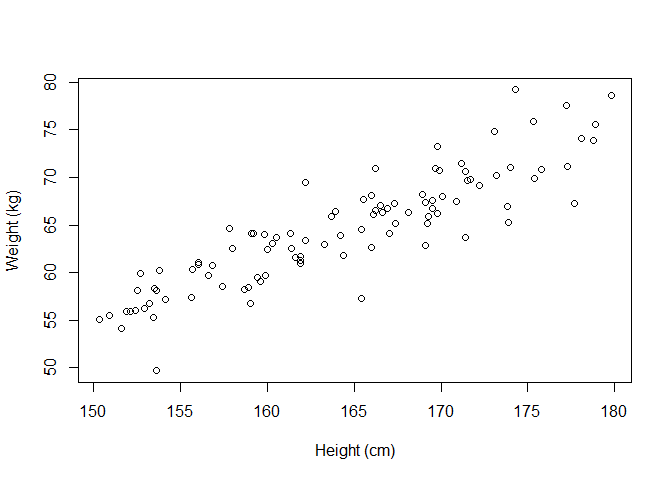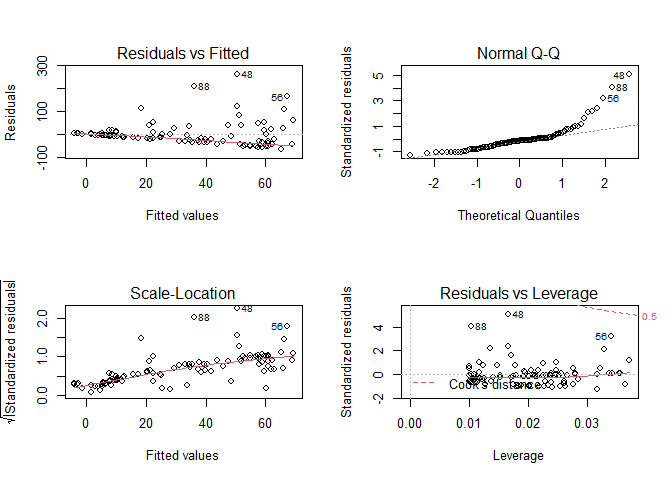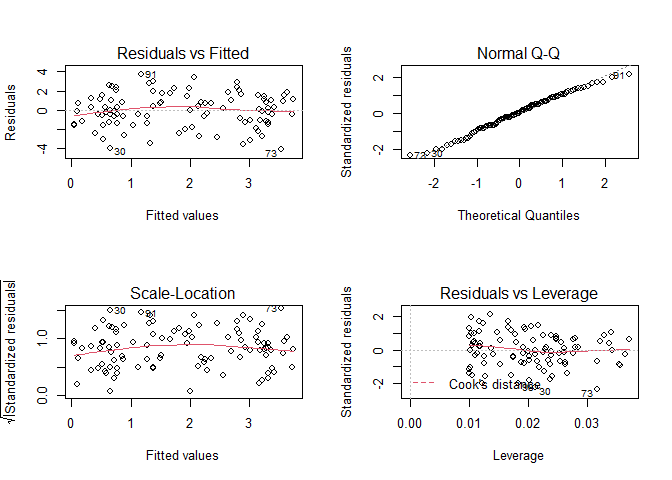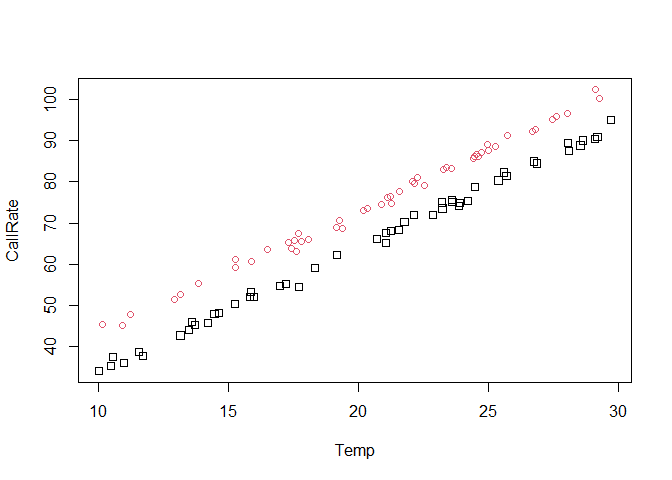# ANCOVA and Linear Models: Example Assignment and Solutions

## Exercise 1

Researchers are interested in the relationship between height in cm and weight in kg. The file weight_height.csv contains the heights in cm and the weights in kg for 100 people.

1. Read in the data and produce a suitably labeled plot of weight (y-axis) against height (x-axis).
df <- read.csv("weight_height.csv")

plot(weight ~ height, data = df,
xlab = "Height (cm)", ylab = "Weight (kg)")1. Fit a linear model to the data to regress y (weight in kg) on x (height in cm). Reproduce and interpret the summary table of the linear model in R, including the equation of the fitted line and the proportion of variability explained by the model.
mod <- lm(weight ~ height, data = df)
summary(mod)
##
## Call:
## lm(formula = weight ~ height, data = df)
##
## Residuals:
##     Min      1Q  Median      3Q     Max
## -7.9750 -1.5062 -0.0422  1.6109  7.9519
##
## Coefficients:
##              Estimate Std. Error t value Pr(>|t|)
## (Intercept) -45.73068    5.79994  -7.885 4.47e-12 ***
## height        0.67113    0.03527  19.031  < 2e-16 ***
## ---
## Signif. codes:  0 '***' 0.001 '**' 0.01 '*' 0.05 '.' 0.1 ' ' 1
##
## Residual standard error: 2.752 on 98 degrees of freedom
## Multiple R-squared:  0.787,  Adjusted R-squared:  0.7849
## F-statistic: 362.2 on 1 and 98 DF,  p-value: < 2.2e-16

Equation of the fitted line: $$\widehat{weight} = -45.73 + 0.67height$$

Multiple R-squared is 0.787, which means that the model explains 78.7% of variability in weight.

1. What is the meaning of the Estimate in the row labeled “height” in the table of part b)?

It means that for every one cm increase in height, the weight is predicted to increase by 0.67 kg on average.

## Exercise 2

In an experiment to investigate the effect of changes in pH on efficiency of an industrial process, the following data were gathered.

pHEfficiency (%)pHEfficency (%)
5.330.27.756.3
8.059.07.555.4
6.037.36.643.3
6.238.05.127.8
6.742.07.044.8
7.452.07.755.1

Two possible models are

Model 1: $$Y_i = \beta_0 + \beta_1X_i + \epsilon_i$$

Model 2: $$Y_i = \beta_0 + \beta_1X_i + \beta_2X_i^2 + \epsilon_i$$

Fit both linear models (using lm2<-lm(Y~X+I(X^2)) for Model 2, or the equivalent statement substituting the names of the variables in your data frame.)

data <- data.frame(pH = c(5.3, 8.0, 6.0, 6.2, 6.7, 7.4, 7.7, 7.5, 6.6, 5.1, 7.0, 7.7),
Efficiency = c(30.2, 59.0, 37.3, 38.0, 42.0, 52.0,
56.3, 55.4, 43.3, 27.8, 44.8, 55.1))

model1 <- lm(Efficiency ~ pH, data = data)
summary(model1)
##
## Call:
## lm(formula = Efficiency ~ pH, data = data)
##
## Residuals:
##     Min      1Q  Median      3Q     Max
## -2.8341 -0.3387  0.2636  0.8577  2.3357
##
## Coefficients:
##             Estimate Std. Error t value Pr(>|t|)
## (Intercept) -28.3889     3.2832  -8.647 5.92e-06 ***
## pH           10.8604     0.4808  22.588 6.51e-10 ***
## ---
## Signif. codes:  0 '***' 0.001 '**' 0.01 '*' 0.05 '.' 0.1 ' ' 1
##
## Residual standard error: 1.53 on 10 degrees of freedom
## Multiple R-squared:  0.9808, Adjusted R-squared:  0.9789
## F-statistic: 510.2 on 1 and 10 DF,  p-value: 6.51e-10
model2 <- lm(Efficiency ~ pH + I(pH^2), data = data)
summary(model2)
##
## Call:
## lm(formula = Efficiency ~ pH + I(pH^2), data = data)
##
## Residuals:
##      Min       1Q   Median       3Q      Max
## -2.10391 -0.62425  0.03311  0.70171  2.33325
##
## Coefficients:
##             Estimate Std. Error t value Pr(>|t|)
## (Intercept)  16.0767    21.7334   0.740   0.4783
## pH           -2.9898     6.7240  -0.445   0.6671
## I(pH^2)       1.0562     0.5118   2.064   0.0691 .
## ---
## Signif. codes:  0 '***' 0.001 '**' 0.01 '*' 0.05 '.' 0.1 ' ' 1
##
## Residual standard error: 1.329 on 9 degrees of freedom
## Multiple R-squared:  0.987,  Adjusted R-squared:  0.9841
## F-statistic: 340.4 on 2 and 9 DF,  p-value: 3.31e-09
1. What are the best fitting models for models 1 and 2?

Model 1: $$\widehat{Efficiency} = -28.39 + 10.86pH$$

Model 2: $$\widehat{Efficiency} = 16.08 - 2.99pH + 1.06pH^2$$

1. What proportion of variability is explained by each model?

Model 1 explains 98.08 % of variability in Efficiency.

Model 2 explains 98.7 % of variability in Efficiency.

1. Use the anova() function (with the names of the two models as two arguments) to assess whether the inclusion of the quadratic term in Model 2 significantly improves the fit of the model and report your findings. Be explicit about exactly which null hypothesis the F-test is testing.
anova(model1, model2)
## Analysis of Variance Table
##
## Model 1: Efficiency ~ pH
## Model 2: Efficiency ~ pH + I(pH^2)
##   Res.Df    RSS Df Sum of Sq      F  Pr(>F)
## 1     10 23.409
## 2      9 15.890  1    7.5198 4.2592 0.06905 .
## ---
## Signif. codes:  0 '***' 0.001 '**' 0.01 '*' 0.05 '.' 0.1 ' ' 1

H0: $$\beta_2 = 0$$

Since p-value = 0.06905 > 0.05, we cannot reject the null hypothesis at 5 % level of significance. The inclusion of the quadratic term in Model 2 does not significantly improve the fit of the model.

## Exercise 3

Read in the csv file “Question3.csv”.

A.df <- read.csv("Question3.csv")
1. Fit a linear model of y against x in the data frame A.df. Generate the standard diagnostic plots and report your findings.
A.lm <- lm(y ~ x, data = A.df)
par(mfrow = c(2, 2))
plot(A.lm)par(mfrow = c(1, 1))

Residuals vs Fitted plot shows that there is a pattern left in the residuals because they are not equally spread around a horizontal line. It means that x and y probably have non-linear relationship.

Normal Q-Q plot shows that residuals deviate severely from the straight line which means that they are not normally distributed.

In Scale-Location plot the red line is not horizontal and residuals spread wider and wider along the x-axis. This indicates that the residuals do not have equal variance (homoscedasticity assumption is violated).

Residuals vs Leverage plot shows that there are no influential cases.

Use an appropriate log transformation and fit a new linear model.

ly <- log(A.df\$y)
A.lm2 <- lm(ly ~ x, data = A.df)
1. What do you see in the diagnostic plots of this new linear model?
par(mfrow = c(2, 2))
plot(A.lm2)par(mfrow = c(1, 1))

Residuals vs Fitted plot does not show any distinctive pattern, which suggests that relationship between log(y) and x is linear.

Normal Q-Q plot shows that residuals follow a straight line well which means they are normally distributed.

Scale-Location plot shows a horizontal line with equally and randomly spread points. This means the assumption of equal variance (homoscedasticity) is satisfied.

Residuals vs Leverage plot shows that there are no influential cases.

1. What is the fitted model for the transformed data (in its log form)?
summary(A.lm2)
##
## Call:
## lm(formula = ly ~ x, data = A.df)
##
## Residuals:
##     Min      1Q  Median      3Q     Max
## -4.0400 -1.2244  0.0409  1.1961  3.7027
##
## Coefficients:
##             Estimate Std. Error t value Pr(>|t|)
## (Intercept)  0.03247    0.33090   0.098    0.922
## x            0.74618    0.11457   6.513 3.18e-09 ***
## ---
## Signif. codes:  0 '***' 0.001 '**' 0.01 '*' 0.05 '.' 0.1 ' ' 1
##
## Residual standard error: 1.749 on 98 degrees of freedom
## Multiple R-squared:  0.3021, Adjusted R-squared:  0.295
## F-statistic: 42.42 on 1 and 98 DF,  p-value: 3.179e-09

Fitted model is $$\widehat{log(y)} = 0.03 + 0.75x$$

1. What is the interpretation of $$e^{\beta_1}$$ in this transformed example?

$$e^{\beta_1}$$ means that an increase in x of 1 unit results in y being multiplied by $$e^{\beta_1} = 2.11$$.

## Exercise 4: ANCOVA example

One use of ANCOVA (ANalysis of COVAriance) is when there is one continuous explanatory variable and one categorical explanatory variable (factor). Potentially, we may wish to fit a separate regression line for each value of the factor. These may or may not be parallel.

Consider the following three models:

Model 1: $$Y_i = \beta_0 + \beta_1X_i + \epsilon_i$$

Model 2: $$Y_i = \beta_0 + \beta_1X_i + \beta_2d + \epsilon_i$$ (parallel lines)

Model 3: $$Y_i = \beta_0 + \beta_1X_i + \beta_2d + \beta_3X_id + \epsilon_i$$ (non-parallel lines)

Suppose the data table looks like this:

XdX.dY
1.4004.6
7.9005.6
4.314.36.2
10.6110.612.4

So the first $$n_1$$ rows all have $$d = 0$$ and the next $$n_2$$ rows all have $$d = 1$$. (The X.d column is obtained by multiplying the X column by the d column and would not normally feature in the data table.)

By considering the intercept and the gradient for the two groups (d=0 and d=1), answer the following two questions.

1. What does the coefficient $$\beta_2$$ correspond to in model 2?

It corresponds to the group with d=1 and means that an intercept for this group is $$\beta_0 + \beta_2$$.

1. What does the coefficient $$\beta_3$$ correspond to in model 3?

It corresponds to the group with d=1 and means that gradient for this group is $$\beta_1 + \beta_3$$.

Read in the file BirdCalls.csv.

BirdCalls <- read.csv("BirdCalls.csv")

The data are from a (simulated) experiment to assess the influence of temperature on call rate for birds of two species. The three columns of the data frame are Temp (Temperature), Species (coded as a 0 or 1) and CallRate (the rate at which the birds call).

1. Fit an overall linear model regressing CallRate on Temp (ignoring the effect of Species) (Model 1 above). Report your findings, including the equation of the fitted line, the goodness of fit of the model and the significance of the parameters in the model.
mod1 <- lm(CallRate ~ Temp, data = BirdCalls)
summary(mod1)
##
## Call:
## lm(formula = CallRate ~ Temp, data = BirdCalls)
##
## Residuals:
##     Min      1Q  Median      3Q     Max
## -6.8980 -4.2658 -0.1974  4.3263  7.1140
##
## Coefficients:
##             Estimate Std. Error t value Pr(>|t|)
## (Intercept)  7.68117    1.78674   4.299 4.05e-05 ***
## Temp         3.04165    0.08432  36.073  < 2e-16 ***
## ---
## Signif. codes:  0 '***' 0.001 '**' 0.01 '*' 0.05 '.' 0.1 ' ' 1
##
## Residual standard error: 4.56 on 98 degrees of freedom
## Multiple R-squared:   0.93,  Adjusted R-squared:  0.9292
## F-statistic:  1301 on 1 and 98 DF,  p-value: < 2.2e-16

Equation of the fitted line is $$\widehat{CallRate} = 7.68 + 3.04Temp$$

The R-squared for the model is 0.93, which means that the model explains 93% of the variability in CallRate.

Both parameters for the Intercept and Temp are statistically significant (their p-values are smaller than 0.01).

1. Use the following code to plot the data with different colours and symbols for the two species and explain why the overall model is inadequate in this case.
plot(CallRate ~ Temp, data = BirdCalls, pch = Species, col = Species+1)The overall model is inadequate in this case because the plot clearly shows that all the points for one of the species are higher than for the other species. An adequate model would be parallel lines model.

1. Fit a model to regress CallRate on both Temp and Species with no interaction (Model 2 above). Generate and interpret appropriate R output, with particular focus on the interpretation of the parameter estimate for Species in the table.
mod2 <- lm(CallRate ~ Temp + Species, data = BirdCalls)
summary(mod2)
##
## Call:
## lm(formula = CallRate ~ Temp + Species, data = BirdCalls)
##
## Residuals:
##      Min       1Q   Median       3Q      Max
## -2.62449 -0.48942 -0.03041  0.54017  2.45042
##
## Coefficients:
##             Estimate Std. Error t value Pr(>|t|)
## (Intercept)  4.26512    0.40630   10.50   <2e-16 ***
## Temp         2.99318    0.01884  158.86   <2e-16 ***
## Species      8.81838    0.20378   43.27   <2e-16 ***
## ---
## Signif. codes:  0 '***' 0.001 '**' 0.01 '*' 0.05 '.' 0.1 ' ' 1
##
## Residual standard error: 1.017 on 97 degrees of freedom
## Multiple R-squared:  0.9966, Adjusted R-squared:  0.9965
## F-statistic: 1.401e+04 on 2 and 97 DF,  p-value: < 2.2e-16

Equation of the fitted line is $$\widehat{CallRate} = 4.27 + 2.99Temp + 8.82Species$$

The R-squared for the model is 0.9966, which means that the model explains 99.66 % of the variability in CallRate.

All the parameters in the model are statistically significant.

The parameter estimate for Species means that holding temperature fixed, the call rate for Species=1 is expected to be higher by 8.82 compared to Species=0.

1. Fit a model of type 3 above (i.e. including interaction) and report the findings from the ANOVA table. What do you conclude and why?
mod3 <- lm(CallRate ~ Temp * Species, data = BirdCalls)
anova(mod3)
## Analysis of Variance Table
##
## Response: CallRate
##              Df  Sum Sq Mean Sq    F value Pr(>F)
## Temp          1 27055.9 27055.9 26107.6603 <2e-16 ***
## Species       1  1937.2  1937.2  1869.3301 <2e-16 ***
## Temp:Species  1     0.9     0.9     0.8328 0.3637
## Residuals    96    99.5     1.0
## ---
## Signif. codes:  0 '***' 0.001 '**' 0.01 '*' 0.05 '.' 0.1 ' ' 1

The interaction parameter is not statistically significant because its p-value = 0.3637 > 0.05 so we cannot reject the null hypothesis that $$\beta_3 = 0$$. This suggests that the slope parameter for both species is the same.

1. Using the anova command, find the reduction of residual sum of squares for fitting model 3 rather than model 2 and report the significance of the relevant test. How does this relate to the answer to the previous question?
anova(mod2, mod3)
## Analysis of Variance Table
##
## Model 1: CallRate ~ Temp + Species
## Model 2: CallRate ~ Temp * Species
##   Res.Df     RSS Df Sum of Sq      F Pr(>F)
## 1     97 100.350
## 2     96  99.487  1   0.86306 0.8328 0.3637

The residual sum of squares for fitting model 3 rather than model 2 reduces from 100.350 to 99.487 (by 0.863). The p-value for F test is 0.3637, so we cannot reject the null hypothesis that $$\beta_3 = 0$$. This means that interaction term is not statistically significant. As in the previous question, we conclude that the slope parameter for both species is the same.